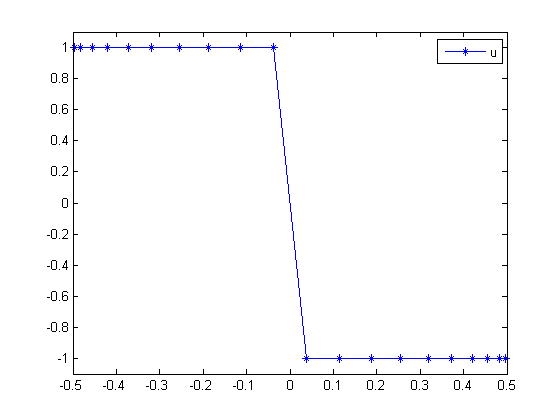98  Simple Bang Bang Problem

Function Space Complementarity Methods for Optimal Control Problems, Dissertation, Martin Weiser

98.1  Problem Description

Find u over t in [-0.5; 0.5 ] to minimize:

J =
 1 2

 1 2
t*u dt

subject to:

 |u| <= 1

Reference: 

98.2  Problem setup

toms t
p = tomPhase('p', t, -0.5, 1, 20);
setPhase(p);
tomStates x
tomControls u

% Initial guess
x0 = {collocate(u == 1-2*(t+0.5))
icollocate(x == 1-2*(t+0.5))};

% Box constraints
cbox = {-1 <= icollocate(x) <= 1
-1 <= collocate(u)  <= 1};

% ODEs and path constraints
ceq = collocate(dot(x) == 0);

% Objective
objective = integrate(t.*u);

98.3  Solve the problem

options = struct;
options.name = 'Simple Bang Bang Problem';
solution = ezsolve(objective, {cbox, ceq}, x0, options);
t = subs(collocate(t),solution);
u = subs(collocate(u),solution);
Problem type appears to be: lp
Starting numeric solver
===== * * * =================================================================== * * *
TOMLAB - Tomlab Optimization Inc. Development license  999001. Valid to 2011-02-05
=====================================================================================
Problem: ---  1: Simple Bang Bang Problem       f_k      -0.250490325030179710
sum(|constr|)      0.000000000000810402
f(x_k) + sum(|constr|)     -0.250490325029369300
f(x_0)      0.000000000000000000

Solver: CPLEX.  EXIT=0.  INFORM=1.
CPLEX Dual Simplex LP solver
Optimal solution found

98.4  Plot result

figure(1);
plot(t,u,'*-');
legend('u');
ylim([-1.1,1.1]);